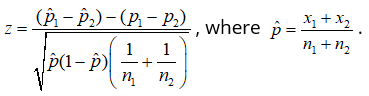##### Statistics All-in-One For Dummies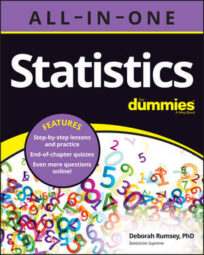Statistics involves a lot of data analysis, and analysis is built with math notation and formulas — but never fear, your cheat sheet is here to help you organize, understand, and remember the notation and formulas so that when it comes to putting them into practice or to the test, you’re ahead of the game!

## 10 tips for the statistically savvy sleuth

Here are some tips on what to do when you encounter statistics in the real world or in the workplace so you can avoid some of the most common statistical mistakes out there. Don’t just read and believe!

• Uncover biased data
• Search for a margin of error
• Identify nonrandom samples
• Notice missing sample sizes
•  Detect misinterpreted correlations
• Point out confounding variables
• Inspect the numbers
• Report selective reporting
• Expose the anecdote

## 10 surefire exam score boosters

Want to get a higher score on your next exam? Put these ten tips into practice to get ahead in your class — and stay ahead.

• Figure out what you don’t know, then do something about it.
• Avoid saying, “Yeah, yeah I know this,” when you, in fact, may not — be active, not passive.
• Make friends with formulas.
• Make an “If-Then” chart. IF my professor asks me X, THEN here’s what I do: Y.
• Figure out exactly what the question is asking.
• Label what you are given in the problem.
• Draw a picture.
• Connect what’s given to what’s being asked, and you can solve the problem.
• Do the math — twice — just to make sure.

## Notation and meanings

Notation is math shorthand for the ideas and concepts used to represent sample and population values. Following are the most common symbols in statistics and what they stand for. Keep this list handy so that you don’t get tripped up by notation.

### Values and statistics from a sample

is the mean of one sample of quantitative data.

s is the standard deviation of one sample of quantitative data.

is the proportion of yeses (successes) in one sample of a binomial data set.

### Values and parameters from a population

X is a random variable that represents any individual value in the population.

x is the value of X for one specific individual from the population.

μx is the mean of the random variable X — also known as the population mean.

σx is the standard deviation of X — also known as the population standard deviation.

p is the proportion of yeses (successes) in the entire binomial population.

### Parameters and statistics regarding the sample mean

is a random variable that represents any sample mean of any sample of size n taken from the population.

μ is the mean of  — also known as the mean of all possible values of μ. Note that μ = μx.

σ is the standard error of  — also known as the standard deviation of all possible values of . Note that σ = σx/√n.

## Distributions

Three distributions are very common in statistics: normal and binomial distributions and sampling distributions. Following are the formulas that are used with each of these three distributions to help solve problems.

Normal: Use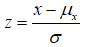Binomial: Mean np; Variance np(1 – p).

Probability: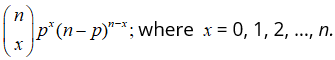Normal Approximation: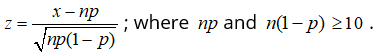Sampling Distribution of :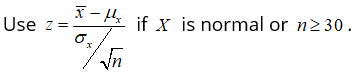## Confidence intervals

Many confidence intervals exist, with the primary purpose of estimating a population parameter. The following list provides a host of formulas for many different confidence interval scenarios from one-sample confidence intervals to those involving two samples from two populations.

One population mean μx with standard deviation known: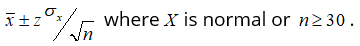One population mean μx with standard deviation unknown: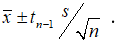One population proportion p with standard deviation unknown:Difference of two population means μxμy with standard deviations known: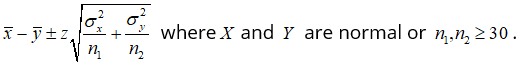Difference of two population means μxμy with standard deviations unknown but equal: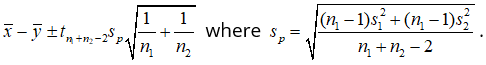Difference of two population means μxμy with standard deviations unknown but unequal: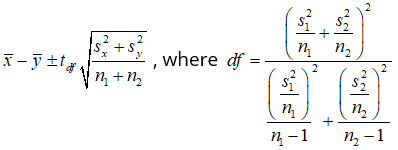Difference of two population proportions p1p2 with standard deviations unknown: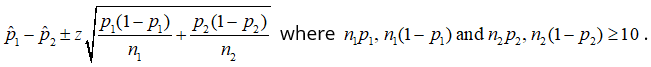## Test statistics for hypothesis tests

Many hypothesis tests exist, with the primary purpose of testing a population parameter. Following are all the test statistics for both one-sample and two-sample hypothesis tests.

One population mean μx with standard deviation known: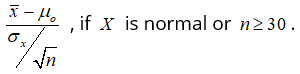One population mean μx with standard deviation unknown: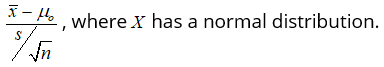One population proportion p: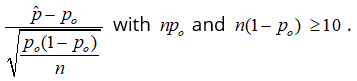Difference of two population means μxμy with standard deviations known: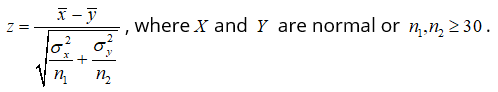Difference of two population means μxμy with standard deviations unknown but equal: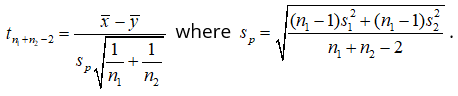Difference of two population means μxμy with standard deviations unknown but unequal: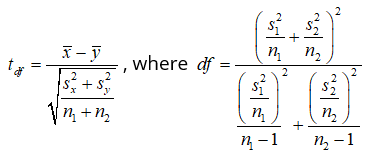Two population proportions: